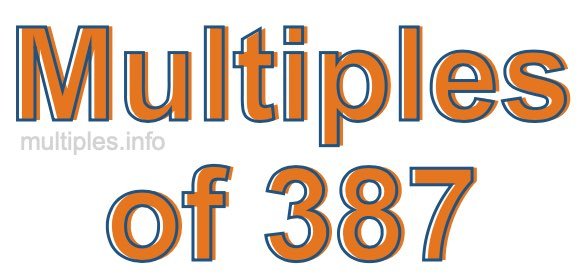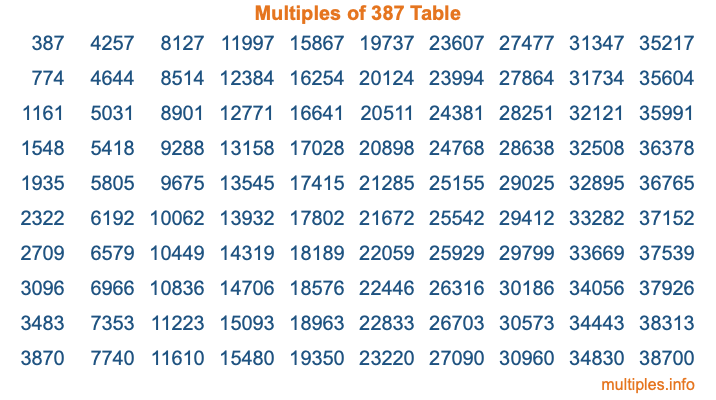Multiples of 387Welcome to the Multiples of 387 page. Here we will first teach you everything you will ever need to know about the multiples of 387, and then give you a study guide summary of everything we taught you to make sure you remember it all. Use this page to look up facts and learn information about the multiples of 387. This page will make you a multiples of three hundred eighty-seven expert!

Definition of Multiples of 387
Multiples of 387 are all the numbers that when divided by 387 equal an integer. Each of the multiples of 387 are called a multiple. A multiple of 387 is created by multiplying 387 by an integer.

Therefore, to create a list of multiples of 387, you start with 1 multiplied by 387, then 2 multiplied by 387, then 3 multiplied by 387, and so on for as long as you want. Thus, the list of the first five multiples of 387 is 387, 774, 1161, 1548, and 1935. To see a larger list of multiples of 387, see the printable image of Multiples of 387 further down on this page. We also have a category where you can choose any nth multiple of 387.

Multiples of 387 Checker
The Multiples of 387 Checker below checks to see if any number of your choice is a multiple of 387. In other words, it checks to see if there is any number (integer) that when multiplied by 387 will equal your number. To do that, we divide your number by 387. If the the quotient is an integer, then your number is a multiple of 387.

Is  a multiple of 387?

Least Common Multiple of 387 and ...
A Least Common Multiple (LCM) is the lowest multiple that two or more numbers have in common. This is also called the smallest common multiple or lowest common multiple and is useful to know when you are adding our subtracting fractions. Enter one or more numbers below (387 is already entered) to find the LCM.

Check out our LCM Calculator if you need more details about the Least Common Multiple or if you need the LCM for different numbers for adding and subtraction fractions.

nth Multiple of 387
As we stated above, 387 is the first multiple of 387, 774 is the second multiple of 387, 1161 is the third multiple of 387, and so on. Enter a number below to find the nth multiple of 387.

th multiple of 387

Multiples of 387 vs Factors of 387
387 is a multiple of 387 and a factor of 387, but that is where the similarities end. All postive multiples of 387 are 387 or greater than 387. All positive factors of 387 are 387 or less than 387.

Below is the beginning list of multiples of 387 and the factors of 387 so you can compare:

Multiples of 387: 387, 774, 1161, 1548, 1935, etc.

Factors of 387: 1, 3, 9, 43, 129, 387

As you can see, the multiples of 387 are all the numbers that you can divide by 387 to get a whole number. The factors of 387, on the other hand, are all the whole numbers that you can multiply by another whole number to get 387.

It's also interesting to note that if a number (x) is a factor of 387, then 387 will also be a multiple of that number (x).

Multiples of 387 vs Divisors of 387
The divisors of 387 are all the integers that 387 can be divided by evenly. Below is a list of the divisors of 387.

Divisors of 387: 1, 3, 9, 43, 129, 387

The interesting thing to note here is that if you take any multiple of 387 and divide it by a divisor of 387, you will see that the quotient is an integer.

Multiples of 387 Table
Below is an image of the first 100 multiples of 387 in a table. The table is in chronological order, column by column. The first column has the first ten multiples of 387, the second column has the next ten multiples of 387, and so on.The Multiples of 387 Table is also referred to as the 387 Times Table or Times Table of 387. You are welcome to print out our table for your studies.

Negative Multiples of 387
Although not often discussed or needed in math, it is worth mentioning that you can make a list of negative multiples of 387 by multiplying 387 by -1, then by -2, then by -3, and so on, to get the following list of negative multiples of 387:

-387, -774, -1161, -1548, -1935, etc.

Multiples of 387 Summary
Below is a summary of important Multiples of 387 facts that we have discussed on this page. To retain the knowledge on this page, we recommend that you read through the summary and explain to yourself or a study partner why they hold true.

There are an infinite number of multiples of 387.

A multiple of 387 divided by 387 will equal a whole number.

387 divided by a factor of 387 equals a divisor of 387.

The nth multiple of 387 is n times 387.

The largest factor of 387 is equal to the first positive multiple of 387.

387 is a multiple of every factor of 387.

387 is a multiple of 387.

A multiple of 387 divided by a divisor of 387 equals an integer.

387 divided by a divisor of 387 equals a factor of 387.

Any integer times 387 will equal a multiple of 387.

Multiples of a Number
Here you can get the multiples of another number, all with the same attention to detail as we did for multiples of 387 on this page.

Multiples of
Multiples of 388
Did you find our page about multiples of three hundred eighty-seven educational? Do you want more knowledge? Check out the multiples of the next number on our list!